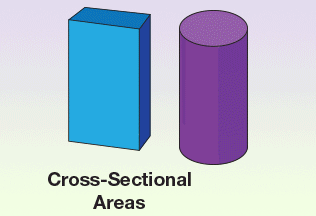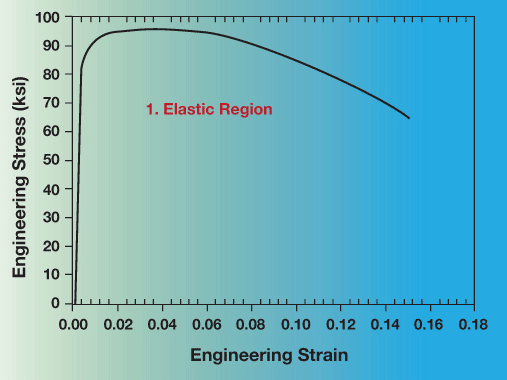Materials Science and EngineeringAlso in this section

# Tensile Test Experiment

One material property that is widely used and recognized is the strength of a material. But what does the word "strength" mean? "Strength" can have many meanings, so let us take a closer look at what is meant by the strength of a material. We will look at a very easy experiment that provides lots of information about the strength or the mechanical behavior of a material, called the tensile test.

The basic idea of a tensile test is to place a sample of a material between two fixtures called "grips" which clamp the material. The material has known dimensions, like length and cross-sectional area. We then begin to apply weight to the material gripped at one end while the other end is fixed. We keep increasing the weight (often called the load or force) while at the same time measuring the change in length of the sample.

## Tensile TestOne can do a very simplified test at home.

If you have a way to hang one end of some material from a solid point that does not move, then you can hang weights on the other end.

Measure the change in length while adding weight until the part begins to stretch and finally breaks.

The result of this test is a graph of load (amount of weight) versus displacement (amount it stretched). Since the amount of weight needed to stretch the material depends on the size of the material (and of course the properties of the material), comparison between materials can be very challenging. The ability to make a proper comparison can be very important to someone designing for structural applications where the material must withstand certain forces.

### Cross-Sectional AreasWe need a way of directly being able to compare different materials, making the “strength” we report independent of the size of the material. We can do that by simply dividing the load applied to the material (the weight or force) by the initial cross-sectional area. We also divide the amount it moves (displacement) by the initial length of the material. This creates what material scientists refer to as engineering stress (load divided by the initial cross-sectional area) and engineering strain (displacement divided by initial length). By looking at the engineering stress-strain response of a material we can compare the strength of different materials, independently of their sizes.

To use the stress-strain response for designing structures, we can divide the load we want by the engineering stress to determine the cross-sectional area needed to be able to hold that load. For example, a 1/8” diameter 4340 steel wire can hold a small car. Again, it is not always that simple. We need to understand the different meanings of “strength” or engineering stress.

Now it gets more complicated. Let us take a look at what is meant by the different strength values and also look at other important properties we can get from this simple test. The easiest way is to examine a graph of engineering stress versus engineering strain. Shown below is a graph of a tensile test for a common steel threaded rod, providing a good example of a general metal tensile test. The units of engineering stress are ksi, which stands for a thousand pounds per square inch. Note the reference to area in the units. The units on strain are of course unitless, since we are dividing distance by distance.## Tensile Tests—Composites

If one pulls on a material until it breaks, one can find out lots of information about the various strengths and mechanical behaviors of a material. In this virtual experiment we will examine the tensile behavior of three different composite fiber materials. They have similar uses but very different properties.

### Experiment

Description: The apparatus pulls on each end of the material until it fractures.

Fiberglass 00:00
Kevlar 01:10
Carbon Fiber 03:09

The video is 5 minutes and 5 seconds with no audio.

Executive Producer Ed Laitila
Host Stephen Forsell
Videographer Britta Lundberg

### Final Data

#### Raw Data for FiberglassThe displacement increases from zero to a little over 5 mm. The load increases almost linearly from 0 to about 12 kN before dropping almost vertically.

#### Corrected Data For FiberglassThe engineering strain increases from zero to about 0.10. The engineering stress increases linearly from zero to about 170 MPa, the fracture strength. The modulus is 1.7 GPa.

#### Corrected Data for KevlarThe engineering strain increases from zero to about 0.11. The engineering stress increases linearly from zero to about 265 MPa, the fracture strength. The modulus is 2.3 GPa.

#### Corrected Data for Carbon FiberThe engineering strain increases from zero to about 0.10. The engineering stress increases linearly from zero to about 580 MPa, the fracture strength. The modulus is 5.7 GPa.

### Conclusions

The carbon fiber composite material has a much higher tensile strength and modulus of elasticity than the other materials. Note they all break in a “brittle” manner, as the curve is linear until it breaks or fractures with no bending of the curve at high loads. Consequently, there is no permanent change in original shape during this test, and hence no ductility.